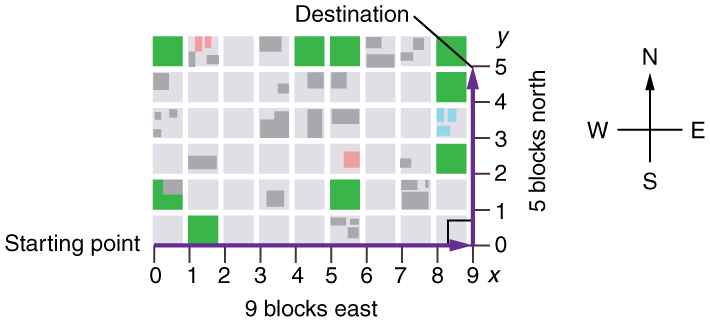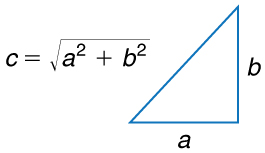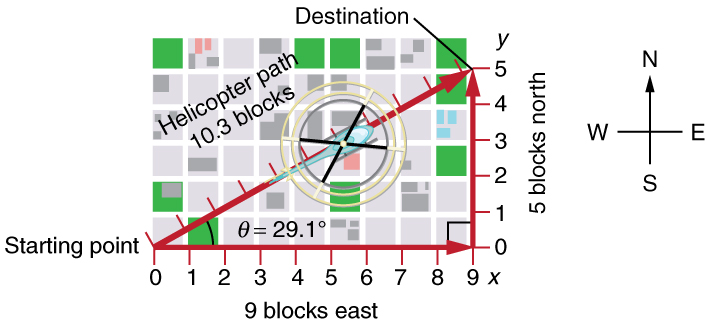# 3.1 Kinematics in two dimensions: an introduction

 Page 1 / 3
• Observe that motion in two dimensions consists of horizontal and vertical components.
• Understand the independence of horizontal and vertical vectors in two-dimensional motion.Walkers and drivers in a city like New York are rarely able to travel in straight lines to reach their destinations. Instead, they must follow roads and sidewalks, making two-dimensional, zigzagged paths. (credit: Margaret W. Carruthers)

## Two-dimensional motion: walking in a city

Suppose you want to walk from one point to another in a city with uniform square blocks, as pictured in [link] .A pedestrian walks a two-dimensional path between two points in a city. In this scene, all blocks are square and are the same size.

The straight-line path that a helicopter might fly is blocked to you as a pedestrian, and so you are forced to take a two-dimensional path, such as the one shown. You walk 14 blocks in all, 9 east followed by 5 north. What is the straight-line distance?

An old adage states that the shortest distance between two points is a straight line. The two legs of the trip and the straight-line path form a right triangle, and so the Pythagorean theorem, , can be used to find the straight-line distance.The Pythagorean theorem relates the length of the legs of a right triangle, labeled a size 12{a} {} and b size 12{b} {} , with the hypotenuse, labeled c size 12{c} {} . The relationship is given by: a 2 +  b 2 =  c 2 size 12{a rSup { size 8{2} }  "+ "b rSup { size 8{2} }  "= "c rSup { size 8{2} } } {} . This can be rewritten, solving for c size 12{A} {} : c  =  a 2 +  b 2 size 12{c" = " sqrt {a rSup { size 8{2} }  "+ "b rSup { size 8{2} } } } {} .

The hypotenuse of the triangle is the straight-line path, and so in this case its length in units of city blocks is , considerably shorter than the 14 blocks you walked. (Note that we are using three significant figures in the answer. Although it appears that “9” and “5” have only one significant digit, they are discrete numbers. In this case “9 blocks” is the same as “9.0 or 9.00 blocks.” We have decided to use three significant figures in the answer in order to show the result more precisely.)The straight-line path followed by a helicopter between the two points is shorter than the 14 blocks walked by the pedestrian. All blocks are square and the same size.

The fact that the straight-line distance (10.3 blocks) in [link] is less than the total distance walked (14 blocks) is one example of a general characteristic of vectors. (Recall that vectors are quantities that have both magnitude and direction.)

As for one-dimensional kinematics, we use arrows to represent vectors. The length of the arrow is proportional to the vector’s magnitude. The arrow’s length is indicated by hash marks in [link] and [link] . The arrow points in the same direction as the vector. For two-dimensional motion, the path of an object can be represented with three vectors: one vector shows the straight-line path between the initial and final points of the motion, one vector shows the horizontal component of the motion, and one vector shows the vertical component of the motion. The horizontal and vertical components of the motion add together to give the straight-line path. For example, observe the three vectors in [link] . The first represents a 9-block displacement east. The second represents a 5-block displacement north. These vectors are added to give the third vector, with a 10.3-block total displacement. The third vector is the straight-line path between the two points. Note that in this example, the vectors that we are adding are perpendicular to each other and thus form a right triangle. This means that we can use the Pythagorean theorem to calculate the magnitude of the total displacement. (Note that we cannot use the Pythagorean theorem to add vectors that are not perpendicular. We will develop techniques for adding vectors having any direction, not just those perpendicular to one another, in Vector Addition and Subtraction: Graphical Methods and Vector Addition and Subtraction: Analytical Methods .)

write an expression for a plane progressive wave moving from left to right along x axis and having amplitude 0.02m, frequency of 650Hz and speed if 680ms-¹
how does a model differ from a theory
what is vector quantity
Vector quality have both direction and magnitude, such as Force, displacement, acceleration and etc.
Besmellah
Is the force attractive or repulsive between the hot and neutral lines hung from power poles? Why?
what's electromagnetic induction
electromagnetic induction is a process in which conductor is put in a particular position and magnetic field keeps varying.
Lukman
wow great
Salaudeen
what is mutual induction?
je
mutual induction can be define as the current flowing in one coil that induces a voltage in an adjacent coil.
Johnson
how to undergo polarization
show that a particle moving under the influence of an attractive force mu/y³ towards the axis x. show that if it be projected from the point (0,k) with the component velocities U and V parallel to the axis of x and y, it will not strike the axis of x unless u>v²k² and distance uk²/√u-vk as origin
show that a particle moving under the influence of an attractive force mu/y^3 towards the axis x. show that if it be projected from the point (0,k) with the component velocities U and V parallel to the axis of x and y, it will not strike the axis of x unless u>v^2k^2 and distance uk^2/√u-k as origin
No idea.... Are you even sure this question exist?
Mavis
I can't even understand the question
yes it was an assignment question "^"represent raise to power pls
Gabriel
Gabriel
An engineer builds two simple pendula. Both are suspended from small wires secured to the ceiling of a room. Each pendulum hovers 2 cm above the floor. Pendulum 1 has a bob with a mass of 10kg . Pendulum 2 has a bob with a mass of 100 kg . Describe how the motion of the pendula will differ if the bobs are both displaced by 12º .
no ideas
Augstine
if u at an angle of 12 degrees their period will be same so as their velocity, that means they both move simultaneously since both both hovers at same length meaning they have the same length
Modern cars are made of materials that make them collapsible upon collision. Explain using physics concept (Force and impulse), how these car designs help with the safety of passengers.
calculate the force due to surface tension required to support a column liquid in a capillary tube 5mm. If the capillary tube is dipped into a beaker of water
find the time required for a train Half a Kilometre long to cross a bridge almost kilometre long racing at 100km/h
method of polarization
Ajayi
What is atomic number?
The number of protons in the nucleus of an atom
Deborah
type of thermodynamics
oxygen gas contained in a ccylinder of volume has a temp of 300k and pressure 2.5×10Nm
why the satellite does not drop to the earth explain
what is a matter
Yinka
what is matter
Yinka
what is matter
Yinka
what is a matter
Yinka
I want the nuclear physics conversation
Mohamed
because space is a vacuum and anything outside the earth 🌎 can not come back without an act of force applied to it to leave the vacuum and fall down to the earth with a maximum force length of 30kcm per second
Clara

#### Get Jobilize Job Search Mobile App in your pocket Now!ByBy Qqq QqqBy Saylor FoundationBy OpenStaxBy Edgar DelgadoBy OpenStaxBy OpenStaxBy Ryan LoweBy Edward BitonBy Szilárd JankóBy Cath Yu